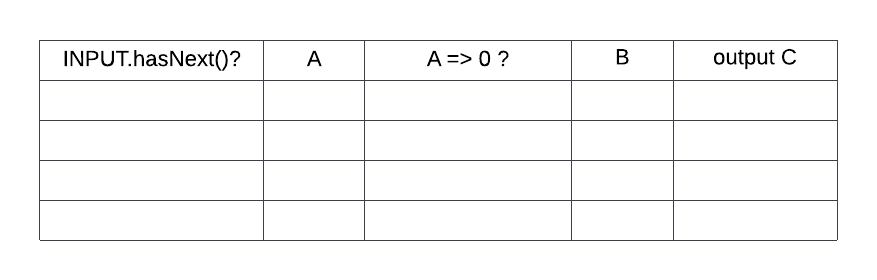Login or Create an Account to view the mark scheme, comment, and add to a test
(a).
Using the table below as a guide, trace the following algorithm by filling out the trace table below row by row (left to right).INPUT = [2, -2, -5, 7, 0] // A collection A=0 B=0 C=0 loop while INPUT.hasNext() A = INPUT.getnext() if (A => 0) then B = INPUT.getnext() end if C = A+B output C end loop
(b).
Explain why binary search is almost always more efficient than linear search on ordered values.

(c).
In the context of programming, define a flag.

(d).
Given two arrays, ALPHA which contains 10 elements and BETA which contains 20 elements, construct an algorithm to determine whether or not ALPHA is a subset of BETA. That is, if all the items in ALPHA are also in BETA then output “SUBSET” otherwise output “NOT SUBSET”


HomeThe Right Test - Fast